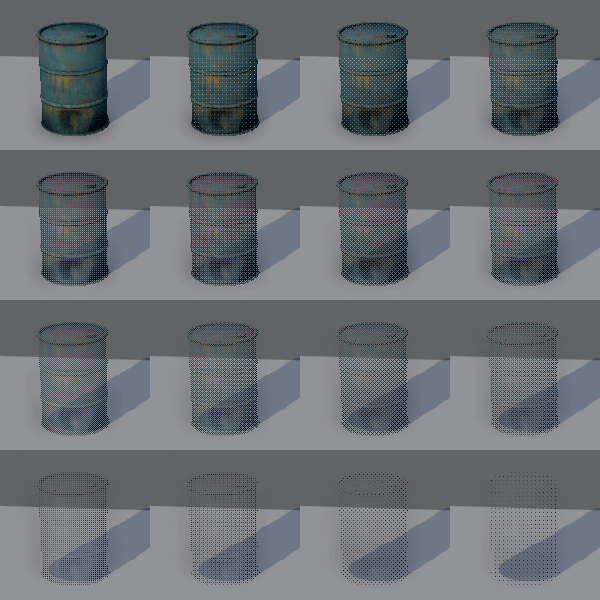While Godot's basic uber shader is great for a number of material scenarios, I've noticed that it doesn't have a feature that I've found really useful in both Blender and Unreal Engine and which I assume wouldn't be too hard to implement. It's called a few things across different software: hashed transparency, dithered opacity, screen door transparency, stipple transparency, or alpha test.

It's basically a way of faking true alpha transparency with 1-bit alphas by using a pattern of dots.

Here's a picture of what I mean:The major advantage of doing transparency like this is you eliminate depth sorting and pixel overdraw issues, something particularly useful for hair and foliage.

Does a shader for this exist already? If not, does anyone have any pointers for writing one?

in Engine

Bizarrely answering my own question here, and I'll likely post this elsewhere for everyone else's use at some point. After much fiddling about, staring blankly at the shader docs, and I've actually come up with something. Go me!

``````// My custom shader for implementing dithered alpha
render_mode blend_mix,depth_draw_opaque,cull_disabled,diffuse_burley,specular_schlick_ggx;

// Texture maps
uniform sampler2D texture_albedo : hint_albedo;
uniform sampler2D texture_normal : hint_normal;

// Parameters
uniform vec4 albedo : hint_color;
uniform float specular = 0.5;
uniform float metallic = 0.0;
uniform float roughness : hint_range(0,1) = 0.5;
uniform float normal_strength : hint_range(0,2) = 1.0;

void fragment() {
vec4 albedo_tex = texture(texture_albedo, UV);
float alpha = albedo_tex.a;

ALBEDO = albedo.rgb * albedo_tex.rgb;
NORMALMAP = texture(texture_normal, UV).rgb;
NORMALMAP_DEPTH = normal_strength;

// Fancy dithered alpha stuff
float opacity = albedo_tex.a;
int x = int(FRAGCOORD.x) % 4;
int y = int(FRAGCOORD.y) % 4;
int index = x + y * 4;
float limit = 0.0;

if (x < 8) {
if (index == 0) limit = 0.0625;
if (index == 1) limit = 0.5625;
if (index == 2) limit = 0.1875;
if (index == 3) limit = 0.6875;
if (index == 4) limit = 0.8125;
if (index == 5) limit = 0.3125;
if (index == 6) limit = 0.9375;
if (index == 7) limit = 0.4375;
if (index == 8) limit = 0.25;
if (index == 9) limit = 0.75;
if (index == 10) limit = 0.125;
if (index == 11) limit = 0.625;
if (index == 12) limit = 1.0;
if (index == 13) limit = 0.5;
if (index == 14) limit = 0.875;
if (index == 15) limit = 0.375;
}
// Is this pixel below the opacity limit? Skip drawing it
if (opacity < limit)
}
``````

I'm sure this could be improved upon; that massive table, for example, could possibly be simplified or replaced with noise but, to channel McCoy out of Star Trek, dammit Jim, I'm a generalist not a technical artist, so I'm not wholly sure what I'd need to be doing there. Suggestions extremely welcome!

by (19 points)

This is already supported in SpatialMaterial for the purposes of fading objects based on distance. Set the distance fade mode to PixelDither or ObjectDither with a minimum and maximum distance:However, we don't have a similar feature for textured materials with an alpha channel yet.

by (12,795 points)

The alpha channel option is more what I'm looking for, though. The distance based fade is less useful.

You can convert any spatial material to a shader material. This way you can at least figure out how it's done. Make sure you have the dithering enabled before converting otherwise that part of the shader will be omitted.

Unfortunately I tried that and it hasn't really lead anywhere. My understanding of the shader ends at precisely the point where it does anything with the dithering. I can just about make out it's performing a test per pixel to see what to render and what to discard but how it does that and how I'd alter it to use an alpha map are completely beyond me at the moment.

The shader in full below, if anyone's interested.

``````shader_type spatial;
render_mode blend_mix,depth_draw_opaque,cull_disabled,diffuse_burley,specular_schlick_ggx;
uniform vec4 albedo : hint_color;
uniform sampler2D texture_albedo : hint_albedo;
uniform float specular;
uniform float metallic;
uniform float roughness : hint_range(0,1);
uniform float point_size : hint_range(0,128);
uniform sampler2D texture_metallic : hint_white;
uniform vec4 metallic_texture_channel;
uniform sampler2D texture_roughness : hint_white;
uniform vec4 roughness_texture_channel;
uniform vec3 uv1_scale;
uniform vec3 uv1_offset;
uniform vec3 uv2_scale;
uniform vec3 uv2_offset;

void vertex() {
UV=UV*uv1_scale.xy+uv1_offset.xy;
}

void fragment() {
vec2 base_uv = UV;
vec4 albedo_tex = texture(texture_albedo,base_uv);
ALBEDO = albedo.rgb * albedo_tex.rgb;
float metallic_tex = dot(texture(texture_metallic,base_uv),metallic_texture_channel);
METALLIC = metallic_tex * metallic;
float roughness_tex = dot(texture(texture_roughness,base_uv),roughness_texture_channel);
ROUGHNESS = roughness_tex * roughness;
SPECULAR = specular;
{
int x = int(FRAGCOORD.x) % 4;
int y = int(FRAGCOORD.y) % 4;
int index = x + y * 4;
float limit = 0.0;

if (x < 8) {
if (index == 0) limit = 0.0625;
if (index == 1) limit = 0.5625;
if (index == 2) limit = 0.1875;
if (index == 3) limit = 0.6875;
if (index == 4) limit = 0.8125;
if (index == 5) limit = 0.3125;
if (index == 6) limit = 0.9375;
if (index == 7) limit = 0.4375;
if (index == 8) limit = 0.25;
if (index == 9) limit = 0.75;
if (index == 10) limit = 0.125;
if (index == 11) limit = 0.625;
if (index == 12) limit = 1.0;
if (index == 13) limit = 0.5;
if (index == 14) limit = 0.875;
if (index == 15) limit = 0.375;
}